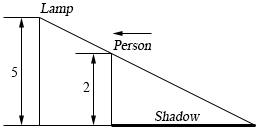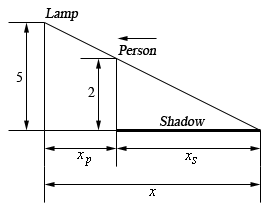Paul's Online Notes
Home / Calculus I / Derivatives / Related Rates
Show Mobile Notice Show All Notes Hide All Notes
Mobile Notice
You appear to be on a device with a "narrow" screen width (i.e. you are probably on a mobile phone). Due to the nature of the mathematics on this site it is best views in landscape mode. If your device is not in landscape mode many of the equations will run off the side of your device (should be able to scroll to see them) and some of the menu items will be cut off due to the narrow screen width.

### Section 3.11 : Related Rates

9. A light is mounted on a wall 5 meters above the ground. A 2 meter tall person is initially 10 meters from the wall and is moving towards the wall at a rate of 0.5 m/sec. After 4 seconds of moving is the tip of the shadow moving (a) towards or away from the person and (b) towards or away from the wall?Show All Solutions Hide All Solutions

Start Solution

Here is a sketch for this situation that will work for both parts so we’ll put it here. Also note that we know that $${x'_p} = - 0.5$$ for both parts.a After 4 seconds of moving is the tip of the shadow towards or away from the person? Show All Steps Hide All Steps
Show Step 2

In this case we want to determine $${x'_s}$$ when $${x_p} = 10 - 4\left( {0.5} \right) = 8$$ (although it will turn out that we simply don’t need this piece of information for this problem….).

We can use the idea of similar triangles to get the following equation.

$\frac{2}{5} = \frac{{{x_s}}}{x} = \frac{{{x_s}}}{{{x_p} + {x_s}}}$

If we solve this for $${x_s}$$ we arrive at,

$\begin{array}{lr}{\textstyle{2 \over 5}}\left( {{x_p} + {x_s}} \right) = {x_s} & \\ {\textstyle{2 \over 5}}{x_p} + {\textstyle{2 \over 5}}{x_s} = {x_s} & \hspace{0.5in} \Rightarrow \hspace{0.5in}{x_s} = {\textstyle{2 \over 3}}{x_p}\end{array}$

This equation will work perfectly for us.

Show Step 3

Differentiation with respect to $$t$$ will give us,

${x'_s} = {\textstyle{2 \over 3}}{x'_p}$ Show Step 4

Finishing off this problem is very simple as all we need to do is plug in the known speed.

${x'_s} = {\textstyle{2 \over 3}}\left( { - 0.5} \right) = - {\textstyle{1 \over 3}}$

Because this rate is negative we can see that the tip of the shadow is moving towards the person at a rate of $${\textstyle{1 \over 3}}$$ m/s.

b After 4 seconds of moving is the tip of the shadow towards or away from the wall? Show All Steps Hide All Steps
Show Step 2

In this case we want to determine $$x'$$ and the equation is really simple. All we need is,

$x = {x_p} + {x_s}$ Show Step 3

Differentiation with respect to $$t$$ will give us,

$x' = {x'_p} + {x'_s}$ Show Step 4

Finishing off this problem is very simple as all we need to do is plug in the known speeds and note that we will need to result from the first part here. So we have $${x'_p} = - {\textstyle{1 \over 2}}$$ from the problem statement and $${x'_s} = - {\textstyle{1 \over 3}}$$ from the previous part.

$x' = - {\textstyle{1 \over 2}} + \left( { - {\textstyle{1 \over 3}}} \right) = - {\textstyle{5 \over 6}}$

Because this rate is negative we can see that the tip of the shadow is moving towards the wall at a rate of $${\textstyle{5 \over 6}}$$ m/s.